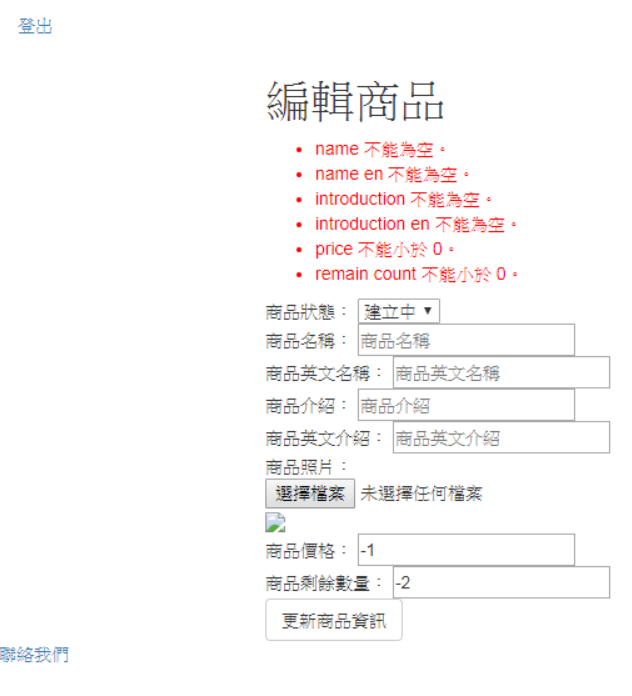#1
Modern Web

## `表單HTTP方法設定`

### `HTTP方法設定`

``````<form action="/merchandise/{{ \$Merchandise->id }}"
method="post"
enctype="multipart/form-data">
<!-- 隱藏方法欄位 -->
{{ method_field('PUT') }}
</form>
``````

``````<input type="hidden" name="_method" value="PUT" />
``````

### `商品資料驗證`

`資料驗證`

app/Http/Controllers/MerchandiseController.php

``````<?PHP
namespace App\Http\Controllers;

use App\Http\Controllers\Controller;
use App\Shop\Entity\Merchandise;
use Validator; //驗證器

class MerchandiseController extends Controller
{
//商品資料更新處理
public function merchandiseItemUpdateProcess(\$merchandise_id)
{
//撈取商品資料
\$Merchandise = Merchandise::findOrFail(\$merchandise_id);
//接收輸入資料
\$input = request()->all();

//驗證規則
\$rules = [
//商品狀態
'status' => [
'required',
'in:C,S' //S前面不能有空格
],
//商品名稱
'name' => [
'required',
'max:80',
],
//商品英文名稱
'name_en' => [
'required',
'max:80',
],
//商品介紹
' introduction' => [
'required',
'max:2000',
],
//商品英文介紹
' introduction_en' => [
'required',
'max:2000',
],
//商品照片
'photo' => [
'file',
'image',
'max:10240', //10 MB
],
//商品價格
'price' => [
'required',
'integer',
'min:0',
],
//商品剩餘數量
'remain_count' => [
'required',
'integer',
'min:0',
],
];

//驗證資料
\$validator = Validator::make(\$input, \$rules);

if(\$validator->fails())
{
//資料驗證錯誤
return redirect('/merchandise/'.\$Merchandise->id.'/edit')
->withErrors(\$validator)
->withInput();
}

var_dump(\$input);
exit();
}
}
?>
``````

`驗證商品照片`

``````//驗證規則
\$rules = [
//商品照片
'photo' => [
'file',
'image',
'max:10240', //10 MB
],
];
``````

### `驗證商品價格及剩餘數量`

``````//驗證規則
\$rules = [
//商品價格
'price' => [
'required',
'integer',
'min:0',
],
//商品剩餘數量
'remain_count' => [
'required',
'integer',
'min:0',
],
];
``````

http://localhost:6943/merchandise/1/edit